1.
Estimate the thermal speed of an oxygen molecule at room temperature.
Hint:
There are NA molecules in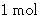Solution:
Since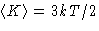, average velocity is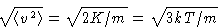An average material scientist might not remember the mass of oxygen molecule, but she/he certainly remembers its molar mass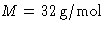. Then m=M/NA. Recalling from Thermo course that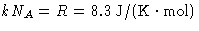, we obtain: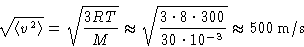This is about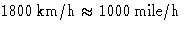2.
Calculate the chemical potential of ideal gas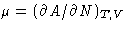.
Solution:
Since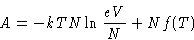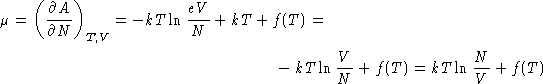Chemical potential of ideal gas is kT log of the concentration (up to a constant)!

3.
Calculate the energy of the ideal gas without internal degrees of freedom (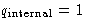). If you are too lazy (or too smart) to calculate it from equation

 E = Nf(T)-NTf'(T) (1)

try to guess the answer. How would you do this?
Solution:
There are two ways to solve this problem.
(a)
Hard way. If,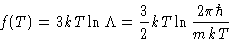and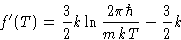so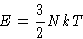(b)
Smart way. If there are no internal degrees of freedom, all the energy is kinetic energy K. This is 3kT/2 per particle, or 3NkT/2 per N particlesNext: Interaction Energy Up: Imperfect Gases and Liquids. Previous: Imperfect Gases and Liquids.

© 1997 Boris Veytsman and Michael Kotelyanskii
Thu Sep 18 22:50:29 EDT 1997